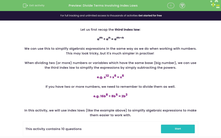# Divide Terms Involving Index Laws

In this worksheet, students will divide terms using index laws to simplify expressions.Key stage:  KS 4

Year:  GCSE

GCSE Subjects:   Maths

GCSE Boards:   Pearson Edexcel, OCR, Eduqas, AQA,

Curriculum topic:   Algebra

Curriculum subtopic:   Notation, Vocabulary and Manipulation Algebraic Expressions

Difficulty level:#### Worksheet Overview

Let us first recap the third index law:

am ÷ an = am-n

We can use this to simplify algebraic expressions in the same way as we do when working with numbers.

This may look tricky, but it's much simpler in practise!

When dividing two (or more) numbers or variables which have the same base (big number), we can use the third index law to simplify the expressions by simply subtracting the powers.

e.g. x12 ÷ x6 = x6

If you have two or more numbers, we need to remember to divide them as well.

e.g. 16b8 ÷ 8b5 = 2b3

In this activity, we will use index laws (like the example above) to simplify algebraic expressions to make them easier to work with.

### What is EdPlace?

We're your National Curriculum aligned online education content provider helping each child succeed in English, maths and science from year 1 to GCSE. With an EdPlace account you’ll be able to track and measure progress, helping each child achieve their best. We build confidence and attainment by personalising each child’s learning at a level that suits them.

Get started